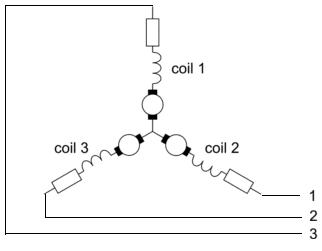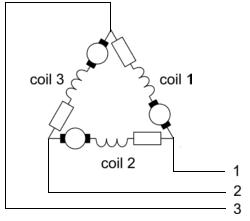# Star Delta Wiring for Alternators

If you plan to make your own three-phase alternator (generator) for a wind turbine or hydro-powered waterwheel then one of the key decisions to make is whether to connect the wires in Star or Delta configuration.

### Star

With star the start of each of the three phases is connected together. Connections are taken from the ends of the three phases to give the three phases.

###Delta

With Delta the starts and ends of the phases are connected. The end of phase 1 is connected to the start of phase 2, the end of phase 2 to the start of phase 3, and the end of phase 3 to the start of phase 1. Connections are taken from the three start-end points to give the three phases.In both cases the three output wires are then connected to Bridge Rectifiers to rectify the generated AC voltage into DC voltage which can then be used to charge a battery bank

### What is the Difference Between Star and Delta

The basic difference between star and delta is that star generates a high voltage at a low current, and delta generates a low voltage at a high current. The total (no load) power generated is the same.

To calculate the output AC voltage and current of a three-phase alternator wired in star or delta it is only necessary to measure the voltage and current of one of the coils. Multiply the voltage of one coil by the number of coils per phase to obtain the phase voltage. The square root of the number of phases (3) = 1.732 can be used to calculate the total outputs with either configuration.

For example, if you have one phase which gives 20 Volts at 12 Amps:
Star – Voltage = 20 * 1.732 = 34.6V, Current is unchanged at 12 Amps.
Delta – Voltage is unchanged at 20 Volts, Current = 12 X 1.732 = 20.8 Amps.

Note that since power is equal to the voltage multiplied by the current, in both cases the power is around 415 Watts in the example above.

We now have an online Star/Delta Wiring Calculator (pictured above) which you can use to calculate the total output voltage and current in both star and delta configurations. You just need to enter the number of coils per phase, number of phases, together with measured values for the current and voltage of one coil.

### Star and Delta in the Real World

Since star and delta wiring both give the same power, why does it matter which is used? Well a wind turbine alternator will generate a higher voltage at lower RPM and will therefore start charging the battery bank sooner if star is used. An alternator wired in delta requires more effort (wind or water power) to get it turning in the first place compared to star. However at high RPM the voltage generated in star can be too high whereas in delta a good current is generated at a lower voltage.

Ideally an alternator would start off wired in star so it starts spinning easily and the high voltage output would start charging sooner, but then at a certain RPM would switch to Delta to output a big current at a not too high voltage. Switching in this way maximises the amount of power actually sent to the batteries and therefore the overall efficiency of the system. Most large commercial wind turbine generators do exactly this switching from star to delta when optimal to do so. The technology is very simple since it is essentially the same as that used to soft-start motors – starting in star and then switching to delta when everything has got going.

### Should I use Star or Delta in my Renewable Energy Project

If you are making your own wind turbine or water wheel alternator then you are usually better off wiring in the Star configuration. As explained above, star is generally more efficient, you will start charging your batteries earlier, and the higher voltages mean you can use thinner (and therefore cheaper) power transmitting cables without incurring large line losses in your system. By using less turns of thicker wire in the coils of the alternator, and wiring in star, you can get a good current while still being able to charge batteries at low RPM.